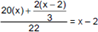# CAT 2017 – Slot 2 – Quantitative Ability – The average height of 22 toddlers increases by 2 inches when two of them leave this group

Q. 1: The average height of 22 toddlers increases by 2 inches when two of them leave this group. If the average height of these two toddlers is one-third the average height of the original 22, then the average height, in inches, of the remaining 20 toddlers is
A) 30
B) 28
C) 32
D) 26

Explanation:- Let the average height of the 20 toddlers be x inches. When the 2 toddlers are included, the average of the group comes down by 2, i.e. it becomes x – 2. Also, given, that the average height of the 2 toddlers is one-third of the average height of the 22 toddlers, viz. x – 2.Upon solving, x = 32 inches.

##### Q. 2: The manufacturer of a table sells it to a wholesale dealer at a profit of 10%. The wholesale dealer sells the table to a retailer at a profit of 30%. Finally, the retailer sells it to a customer at a profit of 50%. If the customer pays Rs 4290 for the table, then its manufacturing cost (in Rs) isA) 1500 B) 2000 C) 2500 D) 3000

Explanation:- Let the manufacturing cost of the table be Rs. m. The wholesaler buys it for Rs.1.1m.

The retailer buys it for Rs.(1.1)(1.3)m.

The customer buys it for Rs.(1.1)(1.3)(1.5)m, viz. Rs. 2.145m. Given, 2.145m = 4,290

⇒ m = Rs.2,000

Q. 3: A tank has an inlet pipe and an outlet pipe. If the outlet pipe is closed then the inlet pipe fills the empty tank in 8 hours. If the outlet pipe is open then the inlet pipe fills the empty tank in 10 hours. If only the outlet pipe is open then in how many hours the full tank becomes half-full?
A) 20
B) 30
C) 40
D) 45

Explanation:- The inlet pipe can normally fill the tank in 8 hours, but it takes 10 hours when the outlet pipe is also open. In the two additional hours, the inlet pipe fills 2/8 or 25% more, and the outlet pipe took 10 hours to drain the additional 25%. So, the outlet pipe can drain 25% in 10 hours, and to drain 50%, it’ll take 20 hours.
Alternately, we can assume total capacity of the tank as LCM [8, 10] or 40 litres. We’ll know the filling rates and the emptying rates of both the pipes and we can answer the question based on the same.

Q. 4: Mayank buys some candies for Rs 15 a dozen and an equal number of different candies for Rs 12 a dozen. He sells all for Rs 16.50 a dozen and makes a profit of Rs 150. How many dozens of candies did he buy altogether?
A) 50
B) 30
C) 25
D) 45

Explanation:- He buys x dozen candies at Rs.15 a dozen and x more dozen at Rs.12 a dozen, so the average cost per dozen is Rs. (15 + 12)/2 = Rs.13.5 a dozen.

By selling a dozen at Rs.16.5, he’ll make a profit of Rs.3 per dozen. To make an overall profit of Rs.150, he needs to sell 50 dozen.

Q. 5: In a village, the production of food grains increased by 40% and the per capita production of food grains increased by 27% during a certain period. The percentage by which the population of the village increased during the same period is nearest to
A) 16
B) 13
C) 10
D) 7

The total production of food grains (Initial) = t

The total production of food grains (Final) = t’ = 1.4t

Population (Initial) = n

Population (Final) = n’

Per capita food production (Initial) = t/n

Per capita food production (Final) = t’/n’ = 1.4t/n’

1.4t/n’ = t/n

=> n’ = 140n/127

Population increased by [(140n/127 – n)/n] * 100 = 10% (Approximately)

Option C is the right answer.

##### Checkout Other Questions of CAT 2017 Slot 2 Paper:

Verbal Ability :              |   Q.01- Q.06  |  Q.07- Q.12  |  Q.13- Q.18  |  Q.19- Q.21  |  Q.22- Q.24  |  Q.25- Q.29  |  Q.30 – Q.34  |

Logical Reasoning :    |   Q.29 – Q.32  |

Quantitative Aptitude: |

### Free Material Area